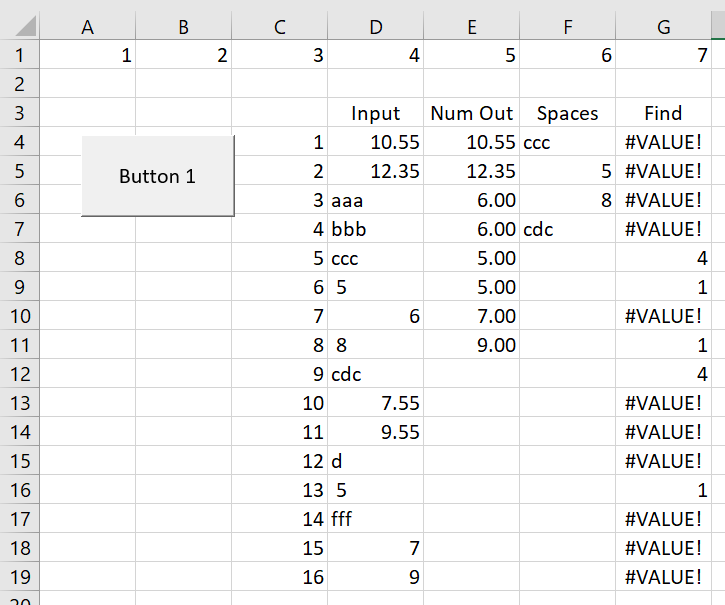# VBA Explanation

This page discusses some of the macros and VBA code for the read pdf file. The VBA and macro discussion covers reading in data, careful error trapping, aggregating European numbers, and other issues. To make the macro and use VBA code for the read pdf analysis, you need to work through each row and possibly column in a file. When reading in the data, I use a FOR/NEXT loop and the CELLS function. In the VBA code you often have to carefully distinguish between numbers and strings. VBA techniques for distinguishing between numbers and columns and working with spaces and strings are  explained with simple and isolated macro examples below.

Before starting on the example, I have posted the read pdf files for you to downolad below.  When you open the file, there are some buttons that allow you to find the various macros.  All of the macros are open source meaning that you can see all of the VBA code. The general way the process works is somewhat like text to columns, but where anything that is a string and not a number is re-combined.

## Error Trapping When Reading Data from Excel

The screenshot and the VBA code below illustrate how to read in separate items from excel.  For this you can use a FOR NEXT loop along with the CELLS function.  In the VBA code the data from Column D are read into the VBA code.  Then, in row 4, all cells that are number and not text are output.  Note that in Column E, only the cells that have numbers are output.  In column F, only the data that include spaces are output.  In column G, I entered the FIND function in excel where the FIND function takes the form FIND(” “,Text in Column D,1) where the 1 at the end stands for the starting number. To make this work you have to do some painful error trapping.The insert of VBA Code below illustrates how you can begin the process by clearing out cells. The statement with the RANGE shows how you can select everything between row 1, column 5 and row 50, column 6.  Then you can do a whole bunch of stuff with this range including clearing the contents as shown in the code below.  You can copy this code and try it yourself.  Below the range, I used the MSGBOX with a defined variable. To do this just start typing the MSGBOX and then after you put the words in double inverted commas, use one of the options.  Then you can test the output of the variables.  In this case, the value of YES is given the number 6.

The file that you can download to work through the VBA code is available to download by pressing the button below.  You can find the VBA code by right clicking on the buttons and then going to assign macro and edit.  Alternatively you can press the Alt and F11 key or the Alt and F8 key.

.

.

```Range(Cells(1, 5), Cells(50, 6)).ClearContents ' Shows how you can refer to cells in a range name
testmsgbox = MsgBox(" Start Test (6-yes) ", vbYesNoCancel) ' Shows how to use the MSGBOX with yes and no
If testmsgbox <> 6 Then Exit Sub ' Demonstrates the result of MSGBOX```

.

.

```For Row = 4 To 19 ' Loop through rows

test_char = Cells(Row, 4) ' Read each item from column 4
re_format(Row) = Cells(Row, 4) ' Define an array variable

If WorksheetFunction.IsNumber(test_char) Then ' Use an excel function
Count = Count + 1
test_num = Val(test_char)
test_num = test_char

Cells(Count + 3, 5) = test_num
End If

.```

.

The video below describes how to make the user form that you can use to allow different options.

## Read PDF Compared with Word Method

There are other methods to convert your pdf file to an excel file.  You can buy some products; there may be other things for free; some may of course be better.  One of the alternatives is to put the PDF into word and then copy it back to excel.  This method is contrasted to the read pdf macro in the videos below.

.